# How to Multiply in Columns with Regrouping

Once students have learned to multiply in columns with no carrying, they move onto multiplying in columns with carrying. This means that the sum of the multiplying numbers is larger than 10, or 100, or 1000, etc., so the resulting 10, 100, 0r 1000 is moved to the column to the left.

Multiplying with regrouping is best explained in an illustrated example.

## Multiplication with carrying

Let’s use this example: 718 x 6

That will look like this in columns: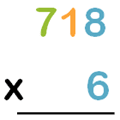When you multiply in columns, you start by multiplying the ones. Like this: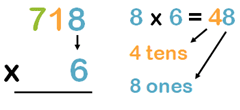The 8 goes in the ones column and the 4 goes in the tens column, like this: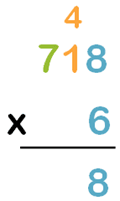Now let’s move onto multiplying the tens column by 6. Don’t forget to add the 4 tens.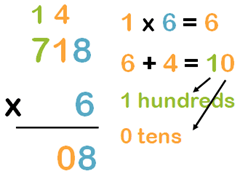See how the 1 hundred moved up to above the 7 hundreds?

Now, let’s multiply the hundreds column with the 6. Don’t forget the add the 1 at the top.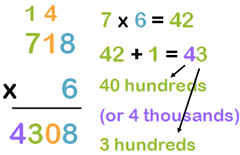The answer is: 718 x 6 = 4,308

## Multiplying with regrouping video

We’ve created a video to demonstrate the above example:

## Worksheets to practice multiplying with carrying

We have worksheets in our grade 3 and grade 4 math sections to practice multiplication with regrouping.

## Grade 3 worksheets for multiplying 1-digit by 3-digit numbers

Students in grade 3 practice multiplying in columns with carrying.## 1-digit by 3-digit multiplication practice for grade 4 students

Grade 4 students work on harder examples of 1-digit by 3-digit multiplication with regrouping.